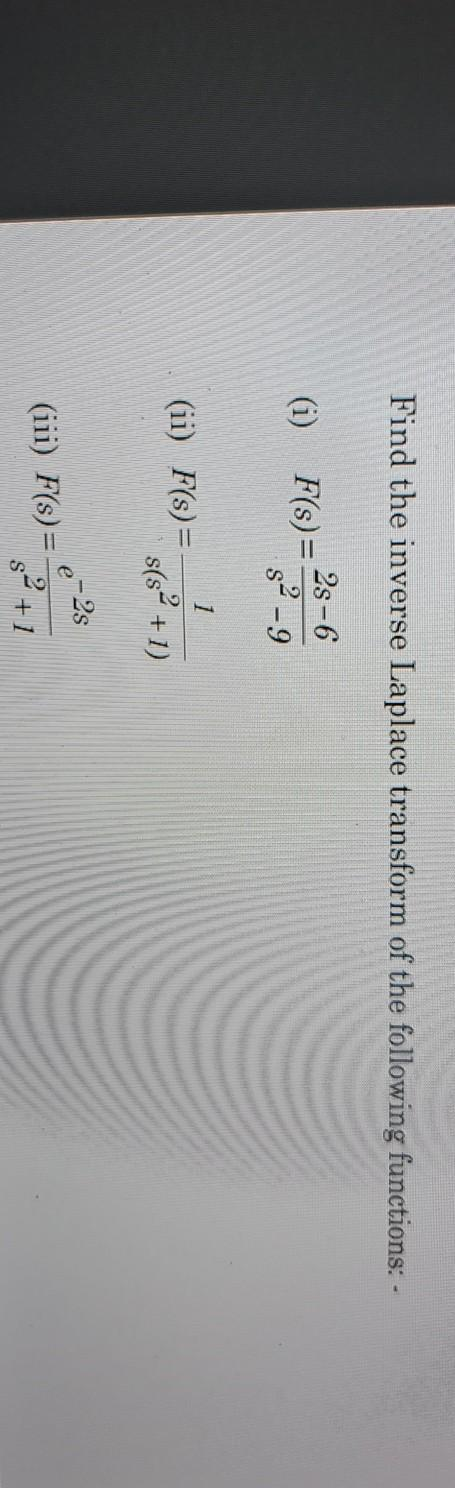### Create an Account

Already have account?

### Forgot Your Password ?

Home / Questions / Find the inverse Laplace transform of the following functions: - (i) 2s -6 F(s) = 8² - 9 1...

# Find the inverse Laplace transform of the following functions: - (i) 2s -6 F(s) = 8² - 9 1 (ii) F(s) = s(sº +1) e-2s (iii) F(s)= \$2+1

Find the inverse Laplace transform of the following functions: - (i) 2s -6 F(s) = 8² - 9 1 (ii) F(s) = s(sº +1) e-2s (iii) F(s)= \$2+1Apr 13 2021 View more View Less

#### Answer (Solved)Subscribe To Get Solution